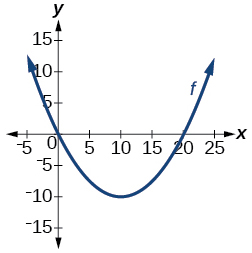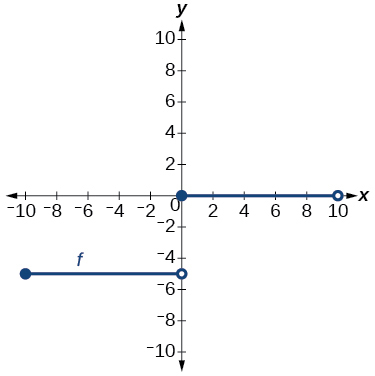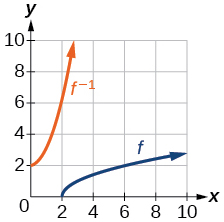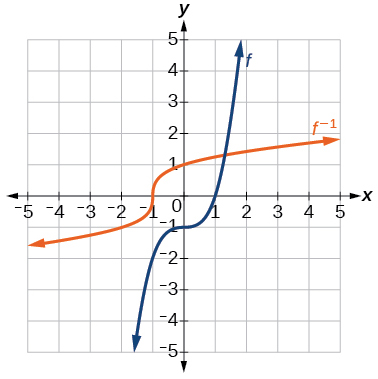# 1.7 Inverse functions  (Page 7/10)

 Page 7 / 10

For the following exercises, use function composition to verify that $\text{\hspace{0.17em}}f\left(x\right)\text{\hspace{0.17em}}$ and $\text{\hspace{0.17em}}g\left(x\right)\text{\hspace{0.17em}}$ are inverse functions.

$f\left(x\right)=\sqrt{x-1}\text{\hspace{0.17em}}$ and $\text{\hspace{0.17em}}g\left(x\right)={x}^{3}+1$

$f\left(x\right)=-3x+5\text{\hspace{0.17em}}$ and $\text{\hspace{0.17em}}g\left(x\right)=\frac{x-5}{-3}$

## Graphical

For the following exercises, use a graphing utility to determine whether each function is one-to-one.

$f\left(x\right)=\sqrt{x}$

one-to-one

$f\left(x\right)=\sqrt{3x+1}$

$f\left(x\right)=-5x+1$

one-to-one

$f\left(x\right)={x}^{3}-27$

For the following exercises, determine whether the graph represents a one-to-one function.not one-to-oneFor the following exercises, use the graph of $\text{\hspace{0.17em}}f\text{\hspace{0.17em}}$ shown in [link] .

Find $\text{\hspace{0.17em}}f\left(0\right).$

$3$

Solve $\text{\hspace{0.17em}}f\left(x\right)=0.$

Find $\text{\hspace{0.17em}}{f}^{-1}\left(0\right).$

$2$

Solve $\text{\hspace{0.17em}}{f}^{-1}\left(x\right)=0.$

For the following exercises, use the graph of the one-to-one function shown in [link] .

Sketch the graph of $\text{\hspace{0.17em}}{f}^{-1}.\text{\hspace{0.17em}}$Find

If the complete graph of $\text{\hspace{0.17em}}f\text{\hspace{0.17em}}$ is shown, find the domain of $\text{\hspace{0.17em}}f.\text{\hspace{0.17em}}$

$\left[2,10\right]$

If the complete graph of $\text{\hspace{0.17em}}f\text{\hspace{0.17em}}$ is shown, find the range of $\text{\hspace{0.17em}}f.$

## Numeric

For the following exercises, evaluate or solve, assuming that the function $\text{\hspace{0.17em}}f\text{\hspace{0.17em}}$ is one-to-one.

If $\text{\hspace{0.17em}}f\left(6\right)=7,\text{\hspace{0.17em}}$ find $\text{\hspace{0.17em}}\text{\hspace{0.17em}}{f}^{-1}\left(7\right).$

$6$

If $\text{\hspace{0.17em}}f\left(3\right)=2,\text{\hspace{0.17em}}$ find $\text{\hspace{0.17em}}{f}^{-1}\left(2\right).$

If $\text{\hspace{0.17em}}{f}^{-1}\left(-4\right)=-8,\text{\hspace{0.17em}}$ find $\text{\hspace{0.17em}}f\left(-8\right).$

$-4$

If $\text{\hspace{0.17em}}{f}^{-1}\left(-2\right)=-1,\text{\hspace{0.17em}}$ find $\text{\hspace{0.17em}}f\left(-1\right).$

For the following exercises, use the values listed in [link] to evaluate or solve.

 $x$ $f\left(x\right)$ 0 8 1 0 2 7 3 4 4 2 5 6 6 5 7 3 8 9 9 1

Find $\text{\hspace{0.17em}}f\left(1\right).$

$0$

Solve $\text{\hspace{0.17em}}f\left(x\right)=3.$

Find $\text{\hspace{0.17em}}{f}^{-1}\left(0\right).$

$\text{\hspace{0.17em}}1\text{\hspace{0.17em}}$

Solve $\text{\hspace{0.17em}}{f}^{-1}\left(x\right)=7.$

Use the tabular representation of $\text{\hspace{0.17em}}f\text{\hspace{0.17em}}$ in [link] to create a table for $\text{\hspace{0.17em}}{f}^{-1}\left(x\right).$

 $x$ 3 6 9 13 14 $f\left(x\right)$ 1 4 7 12 16
 $x$ 1 4 7 12 16 ${f}^{-1}\left(x\right)$ 3 6 9 13 14

## Technology

For the following exercises, find the inverse function. Then, graph the function and its inverse.

$f\left(x\right)=\frac{3}{x-2}$

$f\left(x\right)={x}^{3}-1$

${f}^{-1}\left(x\right)={\left(1+x\right)}^{1/3}$Find the inverse function of $\text{\hspace{0.17em}}f\left(x\right)=\frac{1}{x-1}.\text{\hspace{0.17em}}$ Use a graphing utility to find its domain and range. Write the domain and range in interval notation.

## Real-world applications

To convert from $\text{\hspace{0.17em}}x\text{\hspace{0.17em}}$ degrees Celsius to $\text{\hspace{0.17em}}y\text{\hspace{0.17em}}$ degrees Fahrenheit, we use the formula $\text{\hspace{0.17em}}f\left(x\right)=\frac{9}{5}x+32.\text{\hspace{0.17em}}$ Find the inverse function, if it exists, and explain its meaning.

${f}^{-1}\left(x\right)=\frac{5}{9}\left(x-32\right).\text{\hspace{0.17em}}$ Given the Fahrenheit temperature, $\text{\hspace{0.17em}}x,\text{\hspace{0.17em}}$ this formula allows you to calculate the Celsius temperature.

The circumference $\text{\hspace{0.17em}}C\text{\hspace{0.17em}}$ of a circle is a function of its radius given by $\text{\hspace{0.17em}}C\left(r\right)=2\pi r.\text{\hspace{0.17em}}$ Express the radius of a circle as a function of its circumference. Call this function $\text{\hspace{0.17em}}r\left(C\right).\text{\hspace{0.17em}}$ Find $\text{\hspace{0.17em}}r\left(36\pi \right)\text{\hspace{0.17em}}$ and interpret its meaning.

A car travels at a constant speed of 50 miles per hour. The distance the car travels in miles is a function of time, $\text{\hspace{0.17em}}t,\text{\hspace{0.17em}}$ in hours given by $\text{\hspace{0.17em}}d\left(t\right)=50t.\text{\hspace{0.17em}}$ Find the inverse function by expressing the time of travel in terms of the distance traveled. Call this function $\text{\hspace{0.17em}}t\left(d\right).\text{\hspace{0.17em}}$ Find $\text{\hspace{0.17em}}t\left(180\right)\text{\hspace{0.17em}}$ and interpret its meaning.

$t\left(d\right)=\frac{d}{50},\text{\hspace{0.17em}}$ $t\left(180\right)=\frac{180}{50}.\text{\hspace{0.17em}}$ The time for the car to travel 180 miles is 3.6 hours.

## Functions and Function Notation

For the following exercises, determine whether the relation is a function.

$\left\{\left(a,b\right),\left(c,d\right),\left(e,d\right)\right\}$

function

$\left\{\left(5,2\right),\left(6,1\right),\left(6,2\right),\left(4,8\right)\right\}$

${y}^{2}+4=x,\text{\hspace{0.17em}}$ for $\text{\hspace{0.17em}}x\text{\hspace{0.17em}}$ the independent variable and $\text{\hspace{0.17em}}y\text{\hspace{0.17em}}$ the dependent variable

not a function

Is the graph in [link] a function?

For the following exercises, evaluate the function at the indicated values: $\text{\hspace{0.17em}}\text{\hspace{0.17em}}\text{\hspace{0.17em}}f\left(-3\right);\text{\hspace{0.17em}}\text{\hspace{0.17em}}f\left(2\right);\text{\hspace{0.17em}}\text{\hspace{0.17em}}\text{\hspace{0.17em}}f\left(-a\right);\text{\hspace{0.17em}}\text{\hspace{0.17em}}\text{\hspace{0.17em}}-f\left(a\right);\text{\hspace{0.17em}}\text{\hspace{0.17em}}\text{\hspace{0.17em}}f\left(a+h\right).$

$f\left(x\right)=-2{x}^{2}+3x$

$f\left(-3\right)=-27;$ $f\left(2\right)=-2;$ $f\left(-a\right)=-2{a}^{2}-3a;$
$-f\left(a\right)=2{a}^{2}-3a;$ $f\left(a+h\right)=-2{a}^{2}+3a-4ah+3h-2{h}^{2}$

$f\left(x\right)=2|3x-1|$

For the following exercises, determine whether the functions are one-to-one.

can I get some pretty basic questions
In what way does set notation relate to function notation
Ama
is precalculus needed to take caculus
It depends on what you already know. Just test yourself with some precalculus questions. If you find them easy, you're good to go.
Spiro
the solution doesn't seem right for this problem
what is the domain of f(x)=x-4/x^2-2x-15 then
x is different from -5&3
Seid
how to prroved cos⁴x-sin⁴x= cos²x-sin²x are equal
Don't think that you can.
Elliott
how do you provided cos⁴x-sin⁴x = cos²x-sin²x are equal
What are the question marks for?
Elliott
Someone should please solve it for me Add 2over ×+3 +y-4 over 5 simplify (×+a)with square root of two -×root 2 all over a multiply 1over ×-y{(×-y)(×+y)} over ×y
For the first question, I got (3y-2)/15 Second one, I got Root 2 Third one, I got 1/(y to the fourth power) I dont if it's right cause I can barely understand the question.
Is under distribute property, inverse function, algebra and addition and multiplication function; so is a combined question
Abena
find the equation of the line if m=3, and b=-2
graph the following linear equation using intercepts method. 2x+y=4
Ashley
how
Wargod
what?
John
ok, one moment
UriEl
how do I post your graph for you?
UriEl
it won't let me send an image?
UriEl
also for the first one... y=mx+b so.... y=3x-2
UriEl
y=mx+b you were already given the 'm' and 'b'. so.. y=3x-2
Tommy
Please were did you get y=mx+b from
Abena
y=mx+b is the formula of a straight line. where m = the slope & b = where the line crosses the y-axis. In this case, being that the "m" and "b", are given, all you have to do is plug them into the formula to complete the equation.
Tommy
thanks Tommy
Nimo
0=3x-2 2=3x x=3/2 then . y=3/2X-2 I think
Given
co ordinates for x x=0,(-2,0) x=1,(1,1) x=2,(2,4)
neil
"7"has an open circle and "10"has a filled in circle who can I have a set builder notation
x=-b+_Гb2-(4ac) ______________ 2a
I've run into this: x = r*cos(angle1 + angle2) Which expands to: x = r(cos(angle1)*cos(angle2) - sin(angle1)*sin(angle2)) The r value confuses me here, because distributing it makes: (r*cos(angle2))(cos(angle1) - (r*sin(angle2))(sin(angle1)) How does this make sense? Why does the r distribute once
so good
abdikarin
this is an identity when 2 adding two angles within a cosine. it's called the cosine sum formula. there is also a different formula when cosine has an angle minus another angle it's called the sum and difference formulas and they are under any list of trig identities
strategies to form the general term
carlmark
consider r(a+b) = ra + rb. The a and b are the trig identity.
Mike
How can you tell what type of parent function a graph is ?
generally by how the graph looks and understanding what the base parent functions look like and perform on a graph
William
if you have a graphed line, you can have an idea by how the directions of the line turns, i.e. negative, positive, zero
William
y=x will obviously be a straight line with a zero slope
William
y=x^2 will have a parabolic line opening to positive infinity on both sides of the y axis vice versa with y=-x^2 you'll have both ends of the parabolic line pointing downward heading to negative infinity on both sides of the y axis
William
y=x will be a straight line, but it will have a slope of one. Remember, if y=1 then x=1, so for every unit you rise you move over positively one unit. To get a straight line with a slope of 0, set y=1 or any integer.
Aaron
yes, correction on my end, I meant slope of 1 instead of slope of 0
William
what is f(x)=
I don't understand
Joe
Typically a function 'f' will take 'x' as input, and produce 'y' as output. As 'f(x)=y'. According to Google, "The range of a function is the complete set of all possible resulting values of the dependent variable (y, usually), after we have substituted the domain."
Thomas
Sorry, I don't know where the "Â"s came from. They shouldn't be there. Just ignore them. :-)
Thomas
Darius
Thanks.
Thomas
Â
Thomas
It is the Â that should not be there. It doesn't seem to show if encloses in quotation marks. "Â" or 'Â' ... Â
Thomas
Now it shows, go figure?
Thomas
what is this?
i do not understand anything
unknown
lol...it gets better
Darius
I've been struggling so much through all of this. my final is in four weeks 😭
Tiffany
this book is an excellent resource! have you guys ever looked at the online tutoring? there's one that is called "That Tutor Guy" and he goes over a lot of the concepts
Darius
thank you I have heard of him. I should check him out.
Tiffany
is there any question in particular?
Joe
I have always struggled with math. I get lost really easy, if you have any advice for that, it would help tremendously.
Tiffany
Sure, are you in high school or college?
Darius
Hi, apologies for the delayed response. I'm in college.
Tiffany
how to solve polynomial using a calculatorByByByBy Gerr ZenBy Abishek DevarajByBy